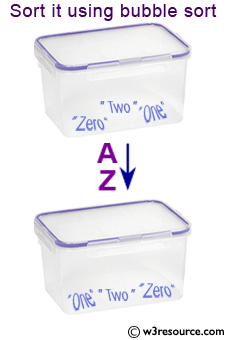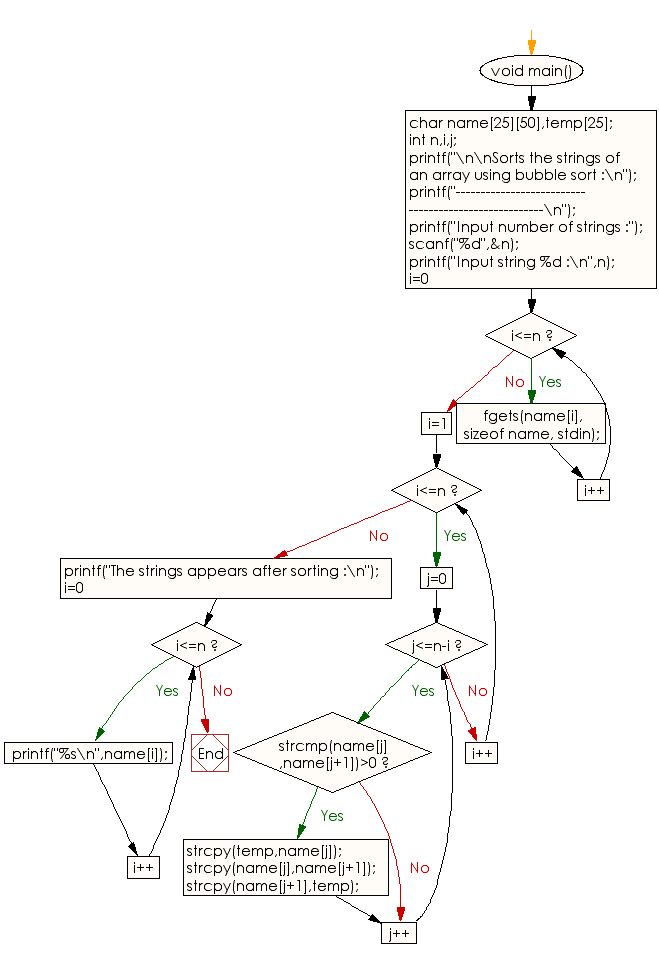﻿ C Program: Sorts the strings of an array using bubble sort - w3resource# C Programming: Sorts the strings of an array using bubble sort

## C String: Exercise-12 with Solution

Write a program in C to read a string through keyboard and sort it using bubble sort.Sample Solution:

C Code:

``````#include <stdio.h>
#include <string.h>
void main()
{
char name,temp;
int n,i,j;

printf("\n\nSorts the strings of an array using bubble sort :\n");
printf("-----------------------------------------------------\n");

printf("Input number of strings :");
scanf("%d",&n);

printf("Input string %d :\n",n);
for(i=0;i<=n;i++)
{

fgets(name[i], sizeof name, stdin);
}
/*Logic Bubble Sort*/

for(i=1;i<=n;i++)
for(j=0;j<=n-i;j++)
if(strcmp(name[j],name[j+1])>0)
{
strcpy(temp,name[j]);
strcpy(name[j],name[j+1]);
strcpy(name[j+1],temp);
}
printf("The strings appears after sorting :\n");
for(i=0;i<=n;i++)
printf("%s\n",name[i]);

}
```
```

Sample Output:

```Sorts the strings of an array using bubble sort :
-----------------------------------------------------
Input number of strings :3
Input string 3 :
zero
one
two
The strings appears after sorting :

one

two

zero
```

Flowchart :C Programming Code Editor:

Improve this sample solution and post your code through Disqus.

What is the difficulty level of this exercise?

Test your Programming skills with w3resource's quiz.

﻿

## C Programming: Tips of the Day

Is there a way to specify how many characters of a string to print out using printf()?

The basic way is:

`printf ("Here are the first 8 chars: %.8s\n", "A string that is more than 8 chars");`

The other, often more useful, way is:

`printf ("Here are the first %d chars: %.*s\n", 8, 8, "A string that is more than 8 chars");`

Here, you specify the length as an int argument to printf(), which treats the '*' in the format as a request to get the length from an argument.

You can also use the notation:

```printf ("Here are the first 8 chars: %*.*s\n",
8, 8, "A string that is more than 8 chars");```

This is also analogous to the "%8.8s" notation, but again allows you to specify the minimum and maximum lengths at runtime - more realistically in a scenario like:

`printf("Data: %*.*s Other info: %d\n", minlen, maxlen, string, info);`

The POSIX specification for printf() defines these mechanism

Ref : https://bit.ly/3u32GyO